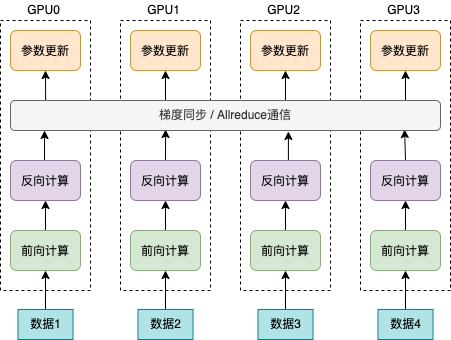# 原理和实践案例¶

## 一、原理介绍¶

1. 各个卡的批处理大小（batch size）和单卡训练保持一致。假设单卡的批处理大小为 `B` ，数据并行训练使用的卡数为 `K` 。那么，数据并行方式下，单次迭代处理的数据量为 `KB` 。在理想情形下，数据并行训练的吞吐量是单卡训练的 `K` 倍。但实际情形下，分布式训练引入了额外的通信和计算开销，如累积各个卡上梯度值并计算平均值。这种额外的通信开销和计算开销通常较小。因此，数据并行训练相比于单卡训练的吞吐量更高，加速比通常略小于 `K`

2. 各个卡的批处理大小总和与单卡训练的批处理大小一致。那么，分布式训练方式下，各个卡的批处理大小为 `B/K` 。因此，分布式训练方式下，每次迭代的时间均明显小于单卡训练，从而在整体上提高训练吞吐量。## 二、动态图使用方法¶

1. 导入依赖包。

2. 初始化分布式环境。

3. 设置模型、优化器。

4. 设置批采样器。

### 2.1 导入依赖包¶

```from paddle.distributed import fleet
```

### 2.2 初始化分布式环境¶

```strategy = fleet.DistributedStrategy()
fleet.init(is_collective=True, strategy=strategy)
```

### 2.3 设置模型、优化器¶

```model = fleet.distributed_model(model)
optimizer = fleet.distributed_optimizer(optimizer)
```

### 2.4 设置批采样器¶

```dataset = RandomDataset(batch_num * batch_size)
sampler = DistributedBatchSampler(dataset,
rank=get_rank(),
batch_size=batch_size,
shuffle=False,
drop_last=True,)
batch_sampler=sampler,
num_workers=1)
```

## 三、动态图运行示例¶

### 3.1 完整训练代码¶

```# -*- coding: UTF-8 -*-
import numpy as np
# 导入必要分布式训练的依赖包
# 导入模型文件

base_lr = 0.1   # 学习率
momentum_rate = 0.9 # 冲量
l2_decay = 1e-4 # 权重衰减

epoch = 10  #训练迭代次数
batch_num = 100 #每次迭代的 batch 数
batch_size = 32 #训练批次大小
class_dim = 102

# 设置数据读取器
class RandomDataset(Dataset):
def __init__(self, num_samples):
self.num_samples = num_samples

def __getitem__(self, idx):
image = np.random.random([3, 224, 224]).astype('float32')
label = np.random.randint(0, class_dim - 1, (1, )).astype('int64')
return image, label

def __len__(self):
return self.num_samples

# 设置优化器
def optimizer_setting(parameter_list=None):
learning_rate=base_lr,
momentum=momentum_rate,
parameters=parameter_list)
return optimizer

# 设置训练函数
def train_model():
# 初始化 Fleet 环境
fleet.init(is_collective=True)

model = ResNet(BottleneckBlock, 50, num_classes=class_dim)

optimizer = optimizer_setting(parameter_list=model.parameters())
# 通过 Fleet API 获取分布式 model，用于支持分布式训练
model = fleet.distributed_model(model)
optimizer = fleet.distributed_optimizer(optimizer)

dataset = RandomDataset(batch_num * batch_size)
# 设置分布式批采样器，用于数据并行训练
sampler = DistributedBatchSampler(dataset, rank=get_rank(),
batch_size=batch_size,shuffle=False, drop_last=True)
batch_sampler=sampler,
num_workers=1)

for eop in range(epoch):
model.train()

img, label = data

out = model(img)

avg_loss.backward()
optimizer.step()

if batch_id % 5 == 0:
print("[Epoch %d, batch %d] loss: %.5f, acc1: %.5f, acc5: %.5f" % (eop, batch_id, avg_loss, acc_top1, acc_top5))
# 启动训练
if __name__ == '__main__':
train_model()
```

### 3.2 运行方式¶

```python -m paddle.distributed.launch --gpus=0,1 --log_dir logs train.py
```